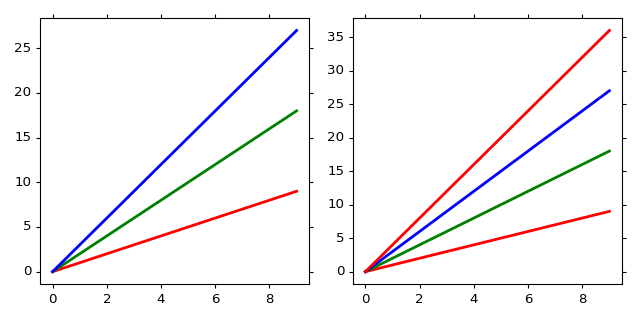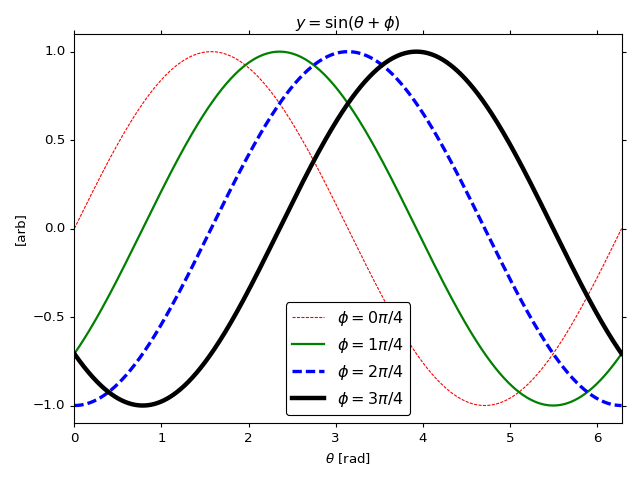# Composable cycles¶

Version: 0.10.0 February 16, 2016

## cycler API¶

 cycler(*args, **kwargs) Create a new Cycler object from a single positional argument, a pair of positional arguments, or the combination of keyword arguments. Cycler(left[, right, op]) Composable cycles concat(left, right) Concatenate two cyclers.

The public API of cycler consists of a class Cycler, a factory function cycler(), and a concatenation function concat(). The factory function provides a simple interface for creating ‘base’ Cycler objects while the class takes care of the composition and iteration logic.

## Cycler Usage¶

### Base¶

A single entry Cycler object can be used to easily cycle over a single style. To create the Cycler use the cycler() function to link a key/style/kwarg to series of values. The key must be hashable (as it will eventually be used as the key in a dict).

In : from __future__ import print_function

In : from cycler import cycler

In : color_cycle = cycler(color=['r', 'g', 'b'])

In : color_cycle
Out: cycler('color', ['r', 'g', 'b'])


The Cycler knows it’s length and keys:

In : len(color_cycle)
Out: 3

In : color_cycle.keys
Out: {'color'}


Iterating over this object will yield a series of dict objects keyed on the label

In : for v in color_cycle:
...:     print(v)
...:
{'color': 'r'}
{'color': 'g'}
{'color': 'b'}


Cycler objects can be passed as the argument to cycler() which returns a new Cycler with a new label, but the same values.

In : cycler(ec=color_cycle)
Out: cycler('ec', ['r', 'g', 'b'])


Iterating over a Cycler results in the finite list of entries, to get an infinite cycle, call the Cycler object (a-la a generator)

In : cc = color_cycle()

In : for j, c in zip(range(5),  cc):
....:     print(j, c)
....:
0 {'color': 'r'}
1 {'color': 'g'}
2 {'color': 'b'}
3 {'color': 'r'}
4 {'color': 'g'}


### Composition¶

A single Cycler can just as easily be replaced by a single for loop. The power of Cycler objects is that they can be composed to easily create complex multi-key cycles.

Equal length Cycler s with different keys can be added to get the ‘inner’ product of two cycles

In : lw_cycle = cycler(lw=range(1, 4))

In : wc = lw_cycle + color_cycle


The result has the same length and has keys which are the union of the two input Cycler‘s.

In : len(wc)
Out: 3

In : wc.keys
Out: {'color', 'lw'}


and iterating over the result is the zip of the two input cycles

In : for s in wc:
....:     print(s)
....:
{'lw': 1, 'color': 'r'}
{'lw': 2, 'color': 'g'}
{'lw': 3, 'color': 'b'}


As with arithmetic, addition is commutative

In : lw_c = lw_cycle + color_cycle

In : c_lw = color_cycle + lw_cycle

In : for j, (a, b) in enumerate(zip(lw_c, c_lw)):
....:    print('({j}) A: {A!r} B: {B!r}'.format(j=j, A=a, B=b))
....:
(0) A: {'lw': 1, 'color': 'r'} B: {'lw': 1, 'color': 'r'}
(1) A: {'lw': 2, 'color': 'g'} B: {'lw': 2, 'color': 'g'}
(2) A: {'lw': 3, 'color': 'b'} B: {'lw': 3, 'color': 'b'}


For convenience, the cycler() function can have multiple key-value pairs and will automatically compose them into a single Cycler via addition

In : wc = cycler(c=['r', 'g', 'b'], lw=range(3))

In : for s in wc:
....:     print(s)
....:
{'c': 'r', 'lw': 0}
{'c': 'g', 'lw': 1}
{'c': 'b', 'lw': 2}


#### Multiplication¶

Any pair of Cycler can be multiplied

In : m_cycle = cycler(marker=['s', 'o'])

In : m_c = m_cycle * color_cycle


which gives the ‘outer product’ of the two cycles (same as itertools.prod() )

In : len(m_c)
Out: 6

In : m_c.keys
Out: {'color', 'marker'}

In : for s in m_c:
....:     print(s)
....:
{'color': 'r', 'marker': 's'}
{'color': 'g', 'marker': 's'}
{'color': 'b', 'marker': 's'}
{'color': 'r', 'marker': 'o'}
{'color': 'g', 'marker': 'o'}
{'color': 'b', 'marker': 'o'}


Note that unlike addition, multiplication is not commutative (like matrices)

In : c_m = color_cycle * m_cycle

In : for j, (a, b) in enumerate(zip(c_m, m_c)):
....:    print('({j}) A: {A!r} B: {B!r}'.format(j=j, A=a, B=b))
....:
(0) A: {'color': 'r', 'marker': 's'} B: {'color': 'r', 'marker': 's'}
(1) A: {'color': 'r', 'marker': 'o'} B: {'color': 'g', 'marker': 's'}
(2) A: {'color': 'g', 'marker': 's'} B: {'color': 'b', 'marker': 's'}
(3) A: {'color': 'g', 'marker': 'o'} B: {'color': 'r', 'marker': 'o'}
(4) A: {'color': 'b', 'marker': 's'} B: {'color': 'g', 'marker': 'o'}
(5) A: {'color': 'b', 'marker': 'o'} B: {'color': 'b', 'marker': 'o'}


#### Integer Multiplication¶

Cycler s can also be multiplied by integer values to increase the length.

In : color_cycle * 2
Out: cycler('color', ['r', 'g', 'b', 'r', 'g', 'b'])

In : 2 * color_cycle
Out: cycler('color', ['r', 'g', 'b', 'r', 'g', 'b'])


#### Concatenation¶

Cycler objects can be concatenated either via the Cycler.concat() method

In : color_cycle.concat(color_cycle)
Out: cycler('color', ['r', 'g', 'b', 'r', 'g', 'b'])


or the top-level concat() function

In : from cycler import concat

In : concat(color_cycle, color_cycle)
Out: cycler('color', ['r', 'g', 'b', 'r', 'g', 'b'])


### Slicing¶

Cycles can be sliced with slice objects

In : color_cycle[::-1]
Out: cycler('color', ['b', 'g', 'r'])

In : color_cycle[:2]
Out: cycler('color', ['r', 'g'])

In : color_cycle[1:]
Out: cycler('color', ['g', 'b'])


to return a sub-set of the cycle as a new Cycler.

### Inspecting the Cycler¶

To inspect the values of the transposed Cycler use the Cycler.by_key method:

In : c_m.by_key()
Out:
{'color': ['r', 'r', 'g', 'g', 'b', 'b'],
'marker': ['s', 'o', 's', 'o', 's', 'o']}


This dict can be mutated and used to create a new Cycler with the updated values

In : bk = c_m.by_key()

In : bk['color'] = ['green'] * len(c_m)

In : cycler(**bk)
Out: (cycler('color', ['green', 'green', 'green', 'green', 'green', 'green']) + cycler('marker', ['s', 'o', 's', 'o', 's', 'o']))


### Examples¶

We can use Cycler instances to cycle over one or more kwarg to plot :

from cycler import cycler
from itertools import cycle

fig, (ax1, ax2) = plt.subplots(1, 2, tight_layout=True,
figsize=(8, 4))
x = np.arange(10)

color_cycle = cycler(c=['r', 'g', 'b'])

for i, sty in enumerate(color_cycle):
ax1.plot(x, x*(i+1), **sty)

for i, sty in zip(range(1, 5), cycle(color_cycle)):
ax2.plot(x, x*i, **sty)from cycler import cycler
from itertools import cycle

fig, (ax1, ax2) = plt.subplots(1, 2, tight_layout=True,
figsize=(8, 4))
x = np.arange(10)

color_cycle = cycler(c=['r', 'g', 'b'])
ls_cycle = cycler('ls', ['-', '--'])
lw_cycle = cycler('lw', range(1, 4))

sty_cycle = ls_cycle * (color_cycle + lw_cycle)

for i, sty in enumerate(sty_cycle):
ax1.plot(x, x*(i+1), **sty)

sty_cycle = (color_cycle + lw_cycle) * ls_cycle

for i, sty in enumerate(sty_cycle):
ax2.plot(x, x*(i+1), **sty)### Persistent Cycles¶

It can be useful to associate a given label with a style via dictionary lookup and to dynamically generate that mapping. This can easily be accomplished using a defaultdict

In : from cycler import cycler as cy

In : from collections import defaultdict

In : cyl = cy('c', 'rgb') + cy('lw', range(1, 4))


To get a finite set of styles

In : finite_cy_iter = iter(cyl)

In : dd_finite = defaultdict(lambda : next(finite_cy_iter))


or repeating

In : loop_cy_iter = cyl()

In : dd_loop = defaultdict(lambda : next(loop_cy_iter))


This can be helpful when plotting complex data which has both a classification and a label

finite_cy_iter = iter(cyl)
styles = defaultdict(lambda : next(finite_cy_iter))
for group, label, data in DataSet:
ax.plot(data, label=label, **styles[group])


which will result in every data with the same group being plotted with the same style.

### Exceptions¶

A ValueError is raised if unequal length Cycler s are added together

In : cycler(c=['r', 'g', 'b']) + cycler(ls=['-', '--'])
---------------------------------------------------------------------------
ValueError                                Traceback (most recent call last)
<ipython-input-47-97d51404b26d> in <module>()
----> 1 cycler(c=['r', 'g', 'b']) + cycler(ls=['-', '--'])

240         if len(self) != len(other):
241             raise ValueError("Can only add equal length cycles, "
--> 242                              "not {0} and {1}".format(len(self), len(other)))
243         return Cycler(self, other, zip)
244

ValueError: Can only add equal length cycles, not 3 and 2


or if two cycles which have overlapping keys are composed

In : color_cycle = cycler(c=['r', 'g', 'b'])

In : color_cycle + color_cycle
---------------------------------------------------------------------------
ValueError                                Traceback (most recent call last)
<ipython-input-49-df525bb380c1> in <module>()
----> 1 color_cycle + color_cycle

241             raise ValueError("Can only add equal length cycles, "
242                              "not {0} and {1}".format(len(self), len(other)))
--> 243         return Cycler(self, other, zip)
244
245     def __mul__(self, other):

/home/tcaswell/.virtualenvs/dd35/lib/python3.5/site-packages/cycler.py in __init__(self, left, right, op)
133             self._right = None
134
--> 135         self._keys = _process_keys(self._left, self._right)
136         self._op = op
137

/home/tcaswell/.virtualenvs/dd35/lib/python3.5/site-packages/cycler.py in _process_keys(left, right)
71     r_key = set(r_peek.keys())
72     if l_key & r_key:
---> 73         raise ValueError("Can not compose overlapping cycles")
74     return l_key | r_key
75

ValueError: Can not compose overlapping cycles


## Motivation¶

When plotting more than one line it is common to want to be able to cycle over one or more artist styles. For simple cases than can be done with out too much trouble:

fig, ax = plt.subplots(tight_layout=True)
x = np.linspace(0, 2*np.pi, 1024)

for i, (lw, c) in enumerate(zip(range(4), ['r', 'g', 'b', 'k'])):
ax.plot(x, np.sin(x - i * np.pi / 4),
label=r'$\phi = {{{0}}} \pi / 4$'.format(i),
lw=lw + 1,
c=c)

ax.set_xlim([0, 2*np.pi])
ax.set_title(r'$y=\sin(\theta + \phi)$')
ax.set_ylabel(r'[arb]')
ax.set_xlabel(r'$\theta$ [rad]')

ax.legend(loc=0)However, if you want to do something more complicated:

fig, ax = plt.subplots(tight_layout=True)
x = np.linspace(0, 2*np.pi, 1024)

for i, (lw, c) in enumerate(zip(range(4), ['r', 'g', 'b', 'k'])):
if i % 2:
ls = '-'
else:
ls = '--'
ax.plot(x, np.sin(x - i * np.pi / 4),
label=r'$\phi = {{{0}}} \pi / 4$'.format(i),
lw=lw + 1,
c=c,
ls=ls)

ax.set_xlim([0, 2*np.pi])
ax.set_title(r'$y=\sin(\theta + \phi)$')
ax.set_ylabel(r'[arb]')
ax.set_xlabel(r'$\theta$ [rad]')

ax.legend(loc=0)the plotting logic can quickly become very involved. To address this and allow easy cycling over arbitrary kwargs the Cycler class, a composable kwarg iterator, was developed.